Courses

# Three Phase Transformers Connections Electrical Engineering (EE) Notes | EduRev

## Electrical Engineering (EE) : Three Phase Transformers Connections Electrical Engineering (EE) Notes | EduRev

The document Three Phase Transformers Connections Electrical Engineering (EE) Notes | EduRev is a part of the Electrical Engineering (EE) Course Electrical Machines.
All you need of Electrical Engineering (EE) at this link: Electrical Engineering (EE)

Three Phase Transformers Connections
We have mentioned earlier that the simplest of the 3 phase transformer will have six windings and twelve terminals.

(Refer Slide Time: 01:04)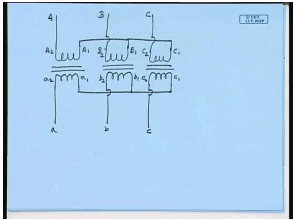Whether they are made from three single phase transformers or built as a single 3 phase unit, there will be at least six windings and twelve terminals. And we have also decided on a terminal convention for them. The HV and LV windings of a particular phase are marked with the same letters. For example, this is called A 2, this is A 1. Then the same instantaneous polarity terminals on the L V side will be called a 2 a 1; capital letter stands for HV winding; small letter stands for LV winding. Similarly, this will be B 2 B 1, b 2 b 1, C 2 C 1, small c 2 small c 1.

Now, there are different types of connections possible with these twelve terminals. For example, the input side can be star connected by connecting the terminals with suffix 1 and supplying power to the rest of the terminal. Similarly, the low voltage type can also be star connected. This will be high voltage phase: A, B, C. This will be low voltage phase: a, b and c. We have also seen that, in this case, the high voltage winding and line voltage phasors will be in phase with the low voltage windings and line voltage phasors respectively.

Line currents on the HV and LV side will also be in phase. In fact, the conversion ratio assuming ideal transformer will be same between the phase voltage of the two sides and the line voltage of the two sides will be same. And that will be equal to the turns ratio of the windings. However, this situation will change. This is also true if both sides are delta connected. However, this situation will change if for example, one side is star connected, the other side is delta connected. Let us see what will be the corresponding conversion ratios.

(Refer Slide Time: 04:42)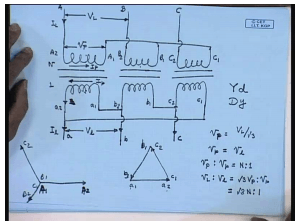So, this is the high voltage side winding – phase A; and the low voltage winding. This is A 2 A 1; this is B 2 B 1; this is C 2 C 1. Similarly, this terminal is a 2, this is a 1; this is b 2, this is b 1; this is c 2, this is c 1. So, let us say the high voltage side is star connected. So, we form the star connection by shorting the terminals A 1, B 1 and C 1 and giving supply to the remaining terminals. So, this is phase A of the high voltage side; this is phase B of the high voltage side; this is phase C. This is the line current I L flowing; and this is the line to line voltage V capital L. And this is the phase voltage V capital P. This is the winding current or phase current I capital P. Similarly, this is the phase current on the LV side I small p; and this is the line current on the LV side I small l.

Now, the low voltage side let us say is delta connected. How do I get this delta connection? Let us say for that, we connect a 1 to b 2, b 1 to c 2 and c 1 to a 2; still get supply from the terminals a 2 b 2 c 2, which constitutes the lines – low voltage lines a, b, c. This is the low voltage line current I small l. And this is the low voltage side line voltage V small l. This is called star-delta connection; that is, the high voltage side star represented by a capital Y and low voltage side is delta represented by a small letter d. Had the high voltage side been delta connected, it would have been written as capital D small y delta-star connection.

Now, let us first try to look at the phasor diagram – the voltage phasor diagram first. Since the basic transformer relation must hold; so this is the phasor let us say B – B 2 B 1; that is, this is the terminal B 2; this is B 1. This is… Let us call it A 1 A 2. This is the phasor C 1 C 2 and this side is the phasor B 1 B 2. So, the corresponding winding voltages will be in phase. So, the voltage V a 1 a 2; this is a 1, this is a 2. Now, on the secondary side, a 1 is connected to terminal b 2. So, this will be the phasor b 1 b 2; and b 1 is connected to c 2. So, this will be the phasor c 2 c 1. So, we see that, voltage small a 2 a 1 is parallel to the voltage V capital A 2 A 1. So, since these two are coupled to each other, similarly for other phasors. So, this is the phasor diagram of a star-delta connected 3 phase transformer.

Now, let us look at the phase voltage, line voltage, phase current and line current transformation ratio. For that, let us assume that, the high voltage side has N number of turns and the low voltage side 1. So, this is the N is to 1 transformer; in which case, we see that, on the high voltage side, the phase voltage V p equal to the line voltage V L by root 3. On the other hand, on the low voltage side, the phase voltage V p equal to the line voltage V l. But, from the transformer relation, V capital P is to V small p is equal to N is to 1. Therefore, V capital L is to V small l equal to root 3 V capital P is to V small p. This will be equal to root 3 N is to 1. So, the phase voltage ratio is same as the turns ratio; whereas, the line voltage ratio is root 3 times the turns ratio.

(Refer Slide Time: 13:50)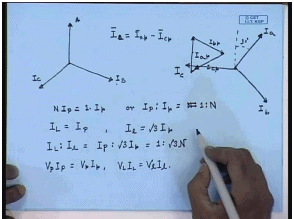Let us look at the currents. So, what will be the current phasor diagram? Again the currents I capital P and I small p will be in phase in this diagram. So, the current flowing from A 2 to A 1 will be in phase with the current flowing from A 1 to… In the LV side in the same phase. So, let us first draw the current from the primary side from the h V side. So, this is let us say the phase current I A, which is same as the line current; this is I B and this is I C. Now, this I A is same as the current flowing through from A 2 to A 1. So, this will be in phase with the current flowing from A 1 to A 2. So, the secondary current flowing through phase A 1 to A 2 will be in phase with this current. So, this is the current I A 1 to A 2, is the phase current I a, which will be in phase with this. Similarly, the phase current I b will be in phase with the high voltage current I B. So, this will be the phase current I b and this will be the phase current I c.

What will be the line currents? Let us say ((Refer Time: 15:36)) I a phase, I b phase, I c phase. What will be the line current? Let us say line current I small a. This is line current I l of the a phase will be phase current I a p minus this current, which is the phase current I c – minus I c p. So, this is I a minus I c p. What will be the I a? This is I c p. So, the phase current I c line phase – I mean the line current I a on the secondary side will be the vector sum of this phasor and the negative of this phasor, which will be somewhat like this. And this angle be 30 degree. This is I small a. Similarly, it can be shown that, the I small b will be 120 degree with respect to this. And I small c will be 120 degree with respect to this. What will be the ratios? Again, if we assume the individual transformers to be ideal; then, we know N times I capital P equal to 1 times I small p or I capital p is to I small p equal to 1 is to N.

Similarly, for star-delta connection, we know the line current I capital L is same as I capital P. On the other hand, line current on the delta side – I small l equal to root 3 times I small p. Therefore, I L is to I l equal to I P is to root 3 I small p. And that is equal to 1 is to root 3 N. Again, it should be noted V P I P equal to V small p I small p as well as V L I L equal to V small l I small l. This relation holds irrespective of the transformer connection. This is because we have assumed the transformer to be ideal. Hence, we have seen that, if one of the sides of the 3 phase transformer is star connected and the other – the high voltage side is star connected, and the low voltage side is delta connected; then, in a nutshell, we can write the relations for the Y d transformers.

(Refer Slide Time: 20:36)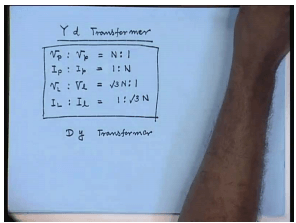Transformation relation for the Y d transformer is V phase is to on the high voltage side to V phase on the low voltage side. This is same as N is to 1. I phase on the high voltage side is to I phase on the low voltage; this is equal to 1 is to N. V line on the high voltage side is to V line on the low voltage side equal to root 3 N is to 1. I line on the high voltage side is to I line on the low voltage side is 1 is to root 3 N. The same similar relation can be written for D y connected transformer. Although star-star, delta-delta, star-delta or delta-star are common connections for 3 phase transformers; there is another interesting connection for the 3 phase transformer. This is called the zig-zag connection.

(Refer Slide Time: 22:45)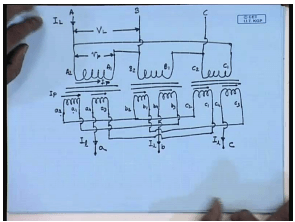For the zig-zag connection, one of the sides, this is let us say the high voltage side of the transformer – A 2 A 1, B 2 B 1, C 2 C 1. And the low voltage side is zig-zag connected. For zig-zag connection, the secondary winding is split into two parts: each low voltage winding is split into two parts. This is small a 2 a 1; this is called a 4 a 3; this is b 2 b 1; this is b 4 b 3; this is c 2 c 1; this is c 4 c 3; indicating all the even number terminals have same instantaneous polarity, and all the odd number terminals have the same instantaneous polarity.

Now, let us assume that, the primary is delta-connected with A 1 connected to B 2, B 1 connected to C 2, and C 1 connected to A 2. And the connections are coming from… External connections are given to A 2, B 2 and C 2. So, this is phase A; this is phase B; this is phase C of the high voltage side. This is the line to line voltage V L – V capital L; this is the phase voltage V p; this is the line current – high voltage side line current I L. Let this be the high voltage side phase current I P.

Similarly, the low voltage side phase current is I small p. This current I will call I a 2 a 1; this is I a 4 a 3; this is I b 2 b 1; that is, from B 2 to B 1. In order to make a zig-zag connection, what is one of these sets of windings are star connected. So, one of them… Let us say a 2, b 2, c 2 is star connected forming the neutral. The terminal a 1 is connected to b 3; and. phase b comes out from b 4. Similarly, the terminal b 1 is connected to c 3; and the line c comes from c 4. Terminal c 1 is connected to a 3 and the a phase connection comes out from a 4. So, these are the line currents I l.

(Refer Slide Time: 30:25)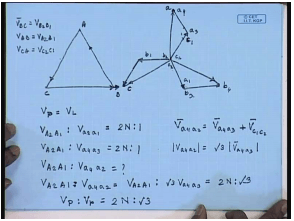First, let us see how the voltage and current phasor diagrams will look like for this kind of a connection. Taking the voltage V B C to be the reference phasor, this is B terminal this is C terminal. So, this is basically the voltage of B 2 with respect to B 1 because B is connected to B 2 and C is connected to B 1. So, this is the voltage V B; this is CA. So, this is BC; this AB; this is CA. So, here we have actually V B C equal to V B 2 B 1; V A B equal to V A 2 A 1; and V C A equal to V C 2 C 1.

Then, on the low voltage side, we see that, the star point is formed by connecting terminals a 2, b 2 and c 2 together. So, the potential V a 1 a 2 will be in phase opposition to voltage V a 2 a 1. That is voltage V A B. So, this will be the phasor V A 2 A 1. Similarly, the phasor B 1, B 2 will be in phasor position to V B 2 B 1 which is V B C. So, this will be the phasor B 1, B 2 and this will be the phasor C 1 C 2. So, this is terminal a 1; this is c 1 and this is b 1.

Now, the phase a obtained by adding the phasor V a 4 a 3 with that of a 3 is connected to c 1. So, phase a obtained by adding phase a 4 a 3, which is parallel to V a b – a 2 a 1 with this phasor. So, this is a 3 a 4. And this is the voltage V a; this is the terminal a. Similarly, phase c is obtained by connecting c 4 c 3 with b 1 b 2, which is parallel to this. Hence, this will be the voltage phasor V c or this is the c phase. And phase b is obtained by connecting b 4 b 3 with a 1 a 2; this is phase b. This is what the voltage phasor diagram will look like. Again we will assume that, the turns ratio is N on the high voltage side is to 0.5 is to 0.5. So, since the primary side is delta connected, V p equal to V L. Also, on the secondary side, let us say, V A 2 A 1 is to V small a 2 small a 1 equal to 2 N is to 1. From this phasor diagram, since a 2 a 1 – the magnitudes of the a 2 a 1 and b 3 b 4… Also, V A 2 A 1 is to V a 4 a 3 is also 2 N is to 1.

Therefore, what is the ratio of V A 2 A 1 is to V a 4 a 2; V a 4 a 2 equal to what? From this phasor diagram, V a 4 a 2 equal to V a 4 a 3 plus V c 1 c 2. But, the magnitude of V c 1 c 2 and V c 3 c 4 are same. And this angle is 120 degree. So, this amplitude is equal… This is equal to… So, mod V a 4 a 2 equal to root 3 times mod V a 4 a 3. Therefore, this will be equal to V a 4 a 2 equal to V A 2 A 1 is to root 3 V a 4 a 3; this will be equal to 2 N is to root 3 or V phase is to V phase equal to 2 N is to root 3. What about the line?

(Refer Slide Time: 39:07)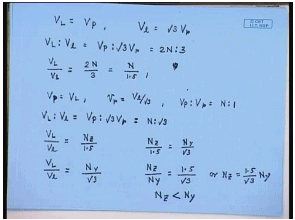The line is simply obtained, because on the high voltage side, V L equal to V P. On the low voltage side, since after all this is star connected, this is incidentally called the zigzag star connection. So, on the low voltage side, V l equal to root 3 V p. Therefore, we have V L is to V l equal to V P is to root 3 V p will be equal to 2 N is to 3. One interesting thing to note here is V L is to V l. In this case, equal to 2 N by 3 or equal to N divided by 1.5. For a star connected system, both side star – there we knew that… Or, if the side was star connected, input side delta connected; output side star connected; in which case, we would have seen it differently. For example, for a delta-star connection, the V p equal to V L.

On the other hand, on the star side, V p equal to V l by root 3. But, for a delta-star connection, V P is to V small p would have been equal to N is to 1. Therefore, V L is to V l would have been V P is to root 3 V p equal to N is to root 3. So, this is the difference. So, suppose I have two transformers: one is delta zig-zag star with turns ratio N z. So, V L is to V l. There is N z is to 1.5. In the other case, I have the same line voltages, but with delta normal star. This is N star by root 3.

Now, if in both cases, the line voltage is on the high voltage and low voltage side are same, then we must have N z by 1.5 equal to N y by root 3 or N z by N y equal to 1.5 by root 3 or N z equal to 1.5 by root 3 into N y. In other words, N z will be less than N y; which shows that, for the same line voltage ratio, if we use a zig-zag star connection on the secondary side, then the number of turns on the HV side can be reduced. So, that gives a cheaper transformer. That is why sometimes it is preferred.

(Refer Slide Time: 44:34)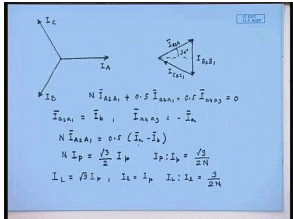Now, let us look at the current phasor diagram. The input side is delta connected. So, let us say this is the line current on the high voltage side – I A; this is I B; and this is I C; I A, I B, I C. From the circuit connection, we have seen that, we can write from here that, line current I A equal to… This current equal to this current plus this current, which is minus of I C. So, it is I A 2 A 1 minus I C 2 C 1. Similarly, line current I B equal to I B 2 B 1 minus I A 2 A 1; and I C equal to I C 2 C 1 minus I B 2 B 1. If we keep this relations in mind, then the phasors: I A 2 A 1, B 2 B 1, C 2 C 1 will look like this. This is I A 2 A 1; this is I B 2 B 1; this is I C 2 C 1. This angle be 30 degree. Now that we have got the winding currents, we can now draw the winding currents on the low voltage side.

Now, the currents on the low voltage side; from this phasor diagram, we find the current I small a equal to… This is the I small a. The current I small a is basically I a 3 a 4, which is same as current a 3 a 4, same as current I c 2 c 1. Now, it has to be seen that, in order to balance mmf, we must have N times I A 2 A 1 plus 0.5 times I a 2 a 1 plus 0.5 times I a 4 a 3. This must be equal to 0. Now, from the phasor diagram, we have seen that, I a 2 a 1. What is I a 2 a 1? I a 2 a 1 is this current flowing through this terminal through this and coming out. So, this is equal to I b.

Similarly, I a 4 a 3 – this we have already seen; this is equal to minus I a. So, we can write N times I A 2 A 1 equal to 0.5 I a minus I b or N times I capital P equal to root 3 by 2 times I small p, because I a and I b are the phase currents of the low voltage sides, which are 120 degree apart. So, I a minus I b is root 3 times the phase current. Hence, we have I P is to I p equal to root 3 by 2 N. But, on the high voltage side, I L equal to root 3 I p; and on the low voltage side, I l equal to I p. Therefore, we have I L is to small I l equal to 3 by 2 N . So, this is for the delta zig-zag star.

(Refer Slide Time: 53:23)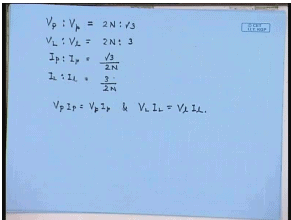Therefore, we can summarize it as V P is to V phase equal to 2 N is to root 3; V L is to V l equal to 2 N is to 3 ((Refer Slide Time: 53:52)). I P is to I p equal to root 3 by 2 N; and I L is to I l equal to 3 by 2 N. Again here also, we see V P I P equal to V p I p; and V L I L equal to V l I l; which is due to the fact that, we have assumed all transformers to be ideal.

Offer running on EduRev: Apply code STAYHOME200 to get INR 200 off on our premium plan EduRev Infinity!

61 docs|20 tests

,

,

,

,

,

,

,

,

,

,

,

,

,

,

,

,

,

,

,

,

,

;